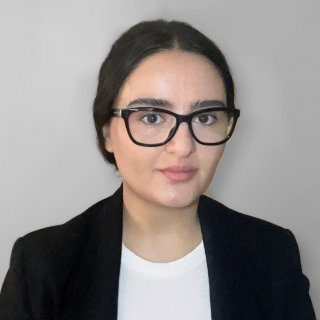EN - USD
Group ClassRussian Math 6th Grade On Russian Math Tutors

Jul, 07 2022

DATES

• Subject - Russian Math

• Time Thu Jul 07, 2022 10:30 - 11:30 PM

• No. of Lessons - 10

• Lesson Days - Thu Sat

• Total Seats - 4

• Price - \$200.00

### Course Details

##### In this class we will learn:

• The concept of denial
• Combined operations with common and decimal fractions.
• Percentages
• Arithmetic mean
• Logic problems
• Rational Numbers
• Sharpen your skills in solving problems based on Mathematical logic and reasoning!

I will guide you in answering all the questions above and more in these lessons. The idea of Build Your Own Math is for you to find solutions by discussion, and trial and error. I am there to present the problem and help you when you need it. And most importantly, ask the question: Why? Whether you get the answer right or wrong, you need to explain how you got there. Then we need to determine if the solution was correct through discussion remember, there can always be more than one way to solve things!